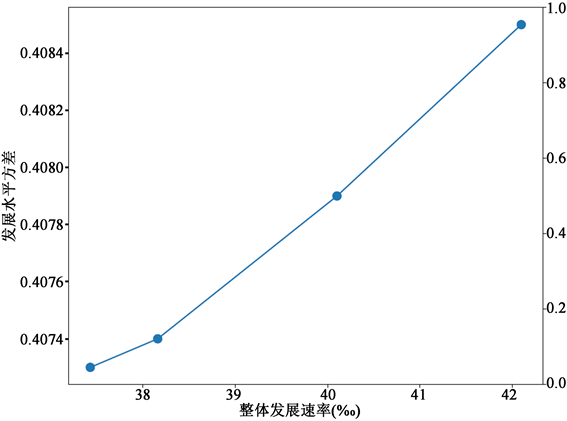#### 期刊菜单

A Method of Allocating Assistance Resources Based on Single-Objective Fractional Model
DOI: 10.12677/MSE.2022.114086, PDF , HTML, XML, 下载: 73  浏览: 128

Abstract: Over the past few decades, China’s economy has grown rapidly and it has gotten rid of absolute poverty in 2020. With the overall economic growth slowing down, promoting balanced development among regions has become an important issue. Under this background, this paper establishes a single-objective fractional programming mathematical model to minimize the regional development difference. Based on this model, a series of quantitative allocation schemes of assistance resources can be obtained. By using the actual economic data of counties and cities in Gansu province to carry out numerical simulation, it is proved that if we distribute the assistance resources based on an allocation scheme in this paper, it can promote the balanced development of the region, and at the same time promote overall development. Therefore, the method of this paper can be applied to the actual non-profit organizations to provide substantive management suggestions, so as to promote balanced and coordinated development of regions.

1. 引言

2. 文献综述

3. 建立模型

${\alpha }_{2}b\le {\sum }_{j\in J}{f}_{j}{p}_{j}\le b$

${t}_{j}={g}_{j}+{g}_{j}{f}_{j}{c}_{j},j\in |J|$

$\left({\sum }_{j\in J}{t}_{j}-{\sum }_{j\in J}{g}_{j}\right)/{\sum }_{j\in J}{t}_{j}\ge lb$

$CV=\frac{\sqrt{{\sum }_{j\in J}{\left({t}_{j}-\mu \right)}^{2}/|J|}}{{\sum }_{j\in J}{t}_{j}/|J|}=\frac{\sqrt{{\sum }_{j\in J}{\left({t}_{j}-\mu \right)}^{2}|J|}}{{\sum }_{j\in J}{t}_{j}}$

$\mu =\frac{{\sum }_{j\in J}{t}_{j}}{|J|}$

$\mathrm{min}\frac{\sqrt{{\sum }_{j\in J}{\left({t}_{j}-\mu \right)}^{2}|J|}}{{\sum }_{j\in J}{t}_{j}}$

s.t

${t}_{j}={g}_{j}+{g}_{j}{f}_{j}{c}_{j},j\in |J|$ (1)

$\left({\sum }_{j\in J}{t}_{j}-{\sum }_{j\in J}{g}_{j}\right)/{\sum }_{j\in J}{g}_{j}\ge lb$ (2)

${\alpha }_{2}b\le {\sum }_{j\in J}{f}_{j}{p}_{j}\le b$ (3)

$0<{\alpha }_{2}\le 1$ (4)

${t}_{j}\ge 0,j\in |J|$ (5)

${f}_{j}\in \left\{0,1\right\},j\in |J|$ (6)

4. 求解方法

$\mathrm{min}\frac{{f}_{1}\left(x\right)}{{f}_{2}\left( x \right)}$

s.t.

$x\in Χ$

$\mathrm{min}{f}_{1}\left(x\right)-q{f}_{2}\left(x\right)$

s.t.

$x\in Χ$

$F\left(q\right)={\mathrm{min}}_{x\in Χ}\left\{{f}_{1}\left(x\right)-q{f}_{2}\left(x\right)\right\}$。当且仅当 ${x}^{\ast }$$P\left({q}^{\ast }\right)$ 的最优解时(此时需满足 $F\left({q}^{\ast }\right)=0$ )， ${x}^{\ast }$ 为问题P的最优解 。因此为了能够找到 ${x}^{\ast }$，首先需要找到 ${q}^{\ast }$，现在引入定理1 ，如下：

1) 当且仅当 $q<{q}^{\ast }$ 时，有 $F\left(q\right)>0$

2) 当且仅当 $q={q}^{\ast }$ 时，有 $F\left(q\right)=0$

3) 当且仅当 $q>{q}^{\ast }$ 时，有 $F\left(q\right)<0$

5. 数值实验

$b={\alpha }_{3}\underset{j\in J}{\sum }{p}_{j}={\alpha }_{3}\underset{j\in J}{\sum }0.1\ast GD{P}_{j}$Table 1. Poverty classifications of counties and cities in Gansu provinceTable 2. The effects of different assistance schemes

· 甘肃各县级市经济发展水平的原始方差为0.4413。在本次数值实验中，得到的4个不同的资源分配方案，均可以不同程度地促进地区发展均衡。其中方案1可以将子区域的经济水平方差降低至0.4073，降低幅度达8.35%；

· 通过设置增长速率约束中的下限，可以发现扶助资金分配方案能帮助地区在保持一定整体发展均速的同时，促进区域发展均衡性。但两者为反相关关系，如图1，即一个资源分配方案最终能够实现的子区域间经济水平差异越小，其对子区域的整体发展增速的提升作用也越小；Figure 1. Trade-off curve between coefficient of variance and overall development rate

· 基于第2点的结论，可以总结到若子区域间均衡发展视为最重要的目标时，得到方案的资金利用率也会较低，因为此时会通过放弃资助部分经济发展水平较高的子区域的方式实现均衡。此时针对未被利用的部分资金，可以通过本模型确定进一步的分配方案，将该部分的剩余资源优先分配给经济发展较差的地区，进而进一步缩小各区域间的经济发展水平。

6. 总结

  薄文广, 安虎森. 我国区域发展思路的演进与未来展望[J]. 南开学报(哲学社会科学版), 2016(3): 115-124.  孙久文, 孙翔宇. 培育经济带: 重塑当代中国区域发展战略[J]. 河北学刊, 2017, 37(2): 114-120.  周长城. 着力补齐全面建成小康社会的短板和弱项[J]. 中国党政干部论坛, 2020(2): 24-28.  姜安印. 论相对贫困的成因、属性及治理之策[J]. 南京农业大学学报(社会科学版), 2021, 21(3): 127-139.  Fudge, M., Ogier, E. and Alexander, K.A. (2021) Emerging Functions of the Wellbeing Concept in Regional Development Scholarship: A Review. Environmental Science & Policy, 115, 143-150. https://doi.org/10.1016/j.envsci.2020.10.005  Shen, H., Teng, F. and Song, J. (2018) Evaluation of Spatial Balance of China’s Regional Development. Sustainability, 10, 3314. https://doi.org/10.3390/su10093314  周绍东. 论基于五大发展理念的全面小康社会建成度[J]. 财会月刊(会计版), 2020(6): 7-11.  Williamson, J.G. (1965) Regional Inequality and the Process of National Development: A Description of the Patterns. Economic Development & Cultural Change, 13, 1-84. https://doi.org/10.1086/450136  Combes, P.P., Lafourcade, M., Thisse, J.F., et al. (2011) The Rise and Fall of Spatial Inequalities in France: A Long-Run Perspective. Explorations in Economic History, 48, 243-271. https://doi.org/10.1016/j.eeh.2010.12.004  Pan, W., Fu, H. and Zheng, P. (2020) Regional Poverty and Inequality in the Xiamen-Zhangzhou-Quanzhou City Cluster in China Based on Npp/Viirs Night-Time Light Imagery. Sustainability, 12, 2547. https://doi.org/10.3390/su12062547  Shu, H. and Xiong, P. (2018) The Gini Coefficient Structure and Its Application for the Evaluation of Regional Balance Development in China. Journal of Cleaner Production, 199, 668-686. https://doi.org/10.1016/j.jclepro.2018.07.224  Li, Y. and Wei, Y.D. (2010) The Spatial-Temporal Hierarchy of Regional Inequality of China. Social Science Electronic Publishing, Rochester.  Tirado, D.A., Diez-Minguela, A. and Martinez-Galarraga, J. (2016) Regional Inequality and Economic Development in Spain, 1860-2010. Journal of Historical Geography, 54, 87-98. https://doi.org/10.1016/j.jhg.2016.09.005  Yi, S, Xiao-Li A. (2018) Application of Threshold Regression Analysis o Study the Impact of Regional Technological Innovation Level of Sustainable Development. Renewable and Sustainable Energy Reviews, 89, 27-32. https://doi.org/10.1016/j.rser.2018.03.005  孙荣臻. 全面建成小康社会与区域经济均衡发展——基于新古典主义区域空间均衡理论[J]. 经济问题探索, 2021(7): 10-19.  Ren, D. (2017) Diversified Monetary Policies Based on the Coordinated Development of Regional Economy. Agro Food Industry Hi Tech, 28, 3415-3418.  Chen, L., Wang, Y., Lai, F., et al. (2016) An Investment Analysis for China’s Sustainable Development Based on Inverse Data Envelopment Analysis. Journal of Cleaner Production, 2016, 1638-1649.  Tang, L. and Wang, D. (2018) Optimization of County-Level Land Resource Allocation through the Improvement of Allocation Efficiency from the Perspective of Sustainable Development. International Journal of Environmental Research & Public Health, 15, 2638. https://doi.org/10.3390/ijerph15122638  赵扶扬, 陈斌开. 土地的区域间配置与新发展格局——基于量化空间均衡的研究[J]. 中国工业经济, 2021(8): 94-113.  Schaible, S. (1976) Fractional Programming. II, on Dinkelbach’s Algorithm. Management Science, 22, 868-873. https://doi.org/10.1287/mnsc.22.8.868  Stancu-Minasian, I. (1997) Fractional Programming: Theory, Methods and Applications. Springer Science & Business Media, Berlin. https://doi.org/10.1007/978-94-009-0035-6  Dinkelbach, W. (1967) On Nonlinear Fractional Programming. Management Science, 13, 492-498. https://doi.org/10.1287/mnsc.13.7.492  程蹊, 陈全功. 较高标准贫困线的确定: 世界银行和美英澳的实践及启示[J]. 贵州社会科学, 2019(6): 141-148.